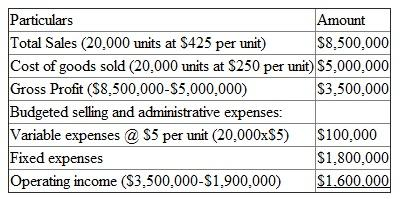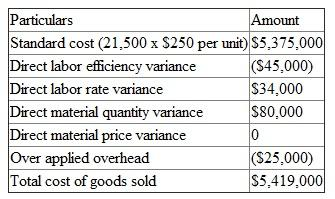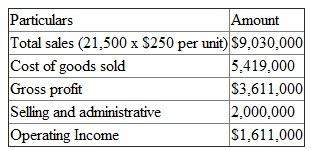# Quiz 25: Rewarding Business Performance

Business

Because the pet shop compensation includes both a fixed (salary) and a variable (commission) component, employees are only paying attention to the portion they can directly affect with their actions. By keeping pet's cages clean and focusing on the tasks necessary to sell the pets, the employees are trying to maximize their compensation. Marsha may want to consider changing the scheme to include careful performance of the other duties before any sales commission can be earned.

(a) B plant produces and sells only one product. A production budget for the coming year based on the available standard, expected sales is 20,000 units, and desired ending inventories is 2000 units (10% of sales). A production budget for the coming year = expected sales + desired ending inventories = 20,000+2,000 = 22,000 units. Thus, production budget of B plant is 22,000 units. (b) Budgeted responsibility income statement for the B plant for the coming year is as follows: B plant Budgeted responsibility income statement Year endingOperating income is calculated by adding variable and fixed expenses and subtract from gross profit. (c) To find out direct labor variances, Standard hourly rate is \$90 per hour. Labor efficiency variance is calculated by subtract actual hours worked from multiplying actual units produced by the plant and standard hours per unit and finally multiply it with standard hourly rate. Labor rate variance is calculated by subtract actual labor costs from multiplying standard rate per hour and actual hours worked. Labor efficiency variance=\$90 per hour (23,000 units x 1.5 hours per unit- 34,000 hours) =\$90 per hour (34,500-34,000) =\$90 per hour (500) =\$45,000 And Labor rate variance = (\$90 per hour x 34,000 hours)-\$3,094,000 = \$3,060,000-\$3,094,000 = (\$34,000) The labor efficiency variance is favorable. It takes only 34,000 hours and save 500 hours. It increases the net income of plant. The labor rate variance is unfavorable due to rate of wages are higher than expected. It decreases the net income. (d) To find out direct materials variances, standard price per pound is \$20 per pound. Materials quality variance is calculated by subtracting actual pounds used from multiple of actual units produced and standard pounds per unit and finally multiple by standard price per pound. Materials quality variance = \$20 per pound (23,000 units x 2-50,000 pounds used) = \$20 per pound (46,000-50,000 pounds) = \$20 per pound (4,000) = (\$80,000) Thus, material quality variance is negative and unfavorable. Material price variance is calculated by subtracting actual direct material costs from multiple of standard price per pound and actual pounds used. Materials price variance = (\$20 per pound x 50,000)-\$1,000,000 = \$1,000,000-\$1,000,000 = \$0 Thus, materials price variance is zero variance. (e) For calculating total overhead applied add fixed and variable cost. Fixed cost for 23,000 units @ \$45 per unit is \$1,035,000 and variable cost for 23,000 units @ \$30 per unit is 690,000. Overhead applied = \$1,035,000+690,000 = \$1,725,000 For calculating actual overhead costs add fixed and variable actual overhead. Actual fixed overhead is \$1,080,000 and actual variable overhead is \$620,000. Total actual overhead = \$1,080,000+\$620,000 = \$1,700,000 Over applied overhead is calculated by subtracting total actual overhead to total overhead applied. Total actual overhead is \$1,700,000 and total overhead applied is \$1,725,000. Over applied overhead = \$1,725,000-\$1,700,000 = \$25,000 After adjustment for over or under applied overhead, the cost of goods sold will be a smaller expense item. (f) For calculating actual plant operating profit for the year, first calculate the cost of goods sold. Cost of goods is calculated by adding Standard cost of units sold and all direct expenses. Calculation of cost of goods soldThe total cost of goods sold is \$5,419,000. For calculating actual plant operating profit subtract cost of goods to total sales and the positive amount is gross profit. Then again subtract selling and administrative expenses to gross profit and the difference amount is operating income. Calculation of Operating IncomeThus, the operating income of B plant is \$1,611,000 (g) The budgeted operating profit of B plant is \$1,600,000 and the actual operating profit of B plant is \$1,611,000. The difference between the budgeted operating profit and the actual operating profit for its first year of operations is \$11,000 (\$1,611,000-\$1,600,000) Actual operating profit is higher than budgeted operating profit. The plant manager efficiently decreased the variable overhead expenses and decreased the price of cost of goods. (h) Return on investment is calculated by dividing Operating income to average total assets. B plant has average investment capital is \$8,050,000 and operating income is \$1,611,000. So,Thus, B plant's ROI is 20 % Return on sales is calculated from dividing Operating income to sales revenue. B plant has sales revenue is \$9,030,000 and operating income is \$1,611,000. So,Thus, B plant ROS is 17.84 % Capital turnover is calculated from Sales revenue to average investment. B plant has sales revenue is \$9,030,000 and average investment is \$8,050,000. So,Thus, B plant CT is 112 % (i) The new equipment return on investment is calculated by dividing Operating income to average total investment in new equipment. New equipment operating earnings is \$108,000 and investment in new equipment is \$600,000. So,Thus, new equipment ROI is 18% The new equipment ROI is only 18% is lower than the B plant return on investment. The addition of new equipment decreases the current ROI and also decreases the bonus of manager. Thus, manager will not undertake the project of new equipment. B plant can use residual income or economic value added or balanced scorecard methods to measure the performances. (j) The B plant manager's should be based on plant ROI after deducting the corporate wide administrative fee. The manager of B plant should be charged for corporate wide administrative fee and should be rewarded based on them being included in the Return on investment calculation.

a. ROI = 12% (\$6,000,000 ÷ \$50,000,000) Residual Income = \$1,000,000 [\$6,000,000 - (\$50,000,000 x 10%)]. b. Both statements are correct. Selling the printers for \$300 each is more profitable than selling them for \$250. If the Printer Division had transferred an additional 10,000 XLC printers to Mail-Order last year, it would have lost \$1,500,000 in contribution margin generated by selling these units to outside customers [Contribution margin per unit (\$250 - \$100) x 10,000 computers]. Thus, its operating earnings would have been only \$4,500,000 (\$6,000,000 actually earned, less \$1,500,000), resulting in a return on investment of only 9% = (\$4,500,000 ÷ \$50,000,000). c. The problem lies in using cost as a transfer price for the output of a profit center. If market value were used as the transfer price, it would be as profitable for the Printer Division to supply printers to Mail-Order as to sell them to outsiders. This should eliminate the interdepartmental friction and allow Wolfe to benefit from selling XLC printers at \$300 instead of \$250. If market price had been used as a transfer price last year, the Printer Division would have recognized \$150 in contribution margin on every unit transferred to the Mail-Order Division (\$250 sales price, less \$100 manufacturing cost). Thus, the Printer Division's contribution margin and operating earnings would have been \$750,000 higher than actually reported. This in turn, would result in a ROI of 13.5% = [(\$6,000,000 + \$750,000) x \$50,000,000] and residual income of \$1,750,000 = (\$6,750,000 - \$5,000,000). d. You have suggested that transfer prices should reflect market prices rather than cost. Had this policy been in effect last year, the residual income of the Printer Division would have been \$750,000 higher than actually reported. As division managers receive a bonus equal to 1% of residual income, Lee may feel that he has lost \$7,500 bonus money due to Wolfe using faulty accounting methods. Actually, using cost as a transfer price has transferred the \$750,000 in contribution margin relating to 5,000 XLC printers from the Printer Division to the Mail-Order Division. Thus, Lee may complain that \$7,500 of his bonus has been paid to Green. Management must decide if some corrective action is necessary.